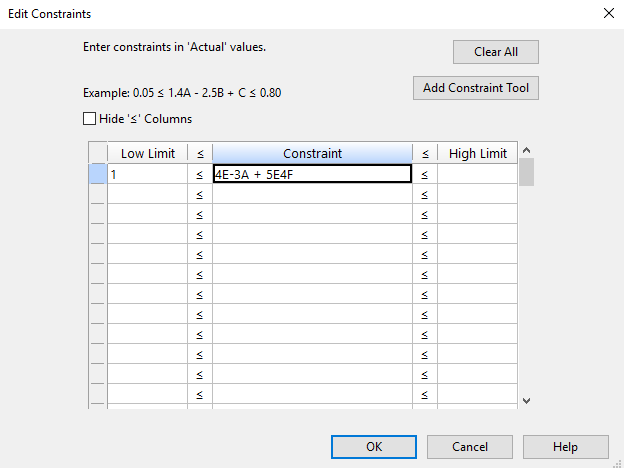# Exponential Notation

Large numbers that are a multiple of 10 raised to an integer power can be written in a convenient compact form using exponential (or engineering) notation. For example, the number 400000, which is 4x10^5, can be written 4E5.

Stat-Ease allows the use of this notation in various places in the program which require a user-inputted equation. For example, the constraint entry tool accepts exponential notation.

The valid forms of exponential notation are as follows:

• Positive Exponents:

• Format: {coefficient}E{power of 10}{factor ID}

• Example: 300 C, where C is a factor, can be written 3E2C in exponential notation.

• Negative Exponents:

• Format: {coefficient}E-{power of 10}{factor ID}

• Example: 0.004 B, where B is a factor, can be written 4E-3B in exponential notation.

Example: The equation “0.004A + 50000F” can be typed as follows in the program:Note

Do not use the + symbol for positive exponents. That is, type 3E2C rather than 3E+2C to represent the number 300. The - symbol is required for negative exponents.